# Intro to Pandas: How to Merge DataFrames

Now that you know about Pandas DataFrames, let's learn how to merge them.
By Boris Delovski • Updated on Jan 6, 2023

In an ideal world, all of the data you need would be stored as a single CSV file, Excel file, or any other format that is supported by Pandas. Unfortunately, in the real world that is often not the case. Most of the time part of the data you need will be stored in multiple different files.

Combining data from these files without loading that data is possible but usually relatively hard to do. Therefore the easiest way to deal with this problem is to just load data from every file into its own separate DataFrame and afterward combine these DataFrames. This procedure of combining data from multiple DataFrames in Pandas is called merging DataFrames.

## How to Merge Pandas DataFrames

To merge DataFrames in Pandas you will use the merge() method. The functionality this method offers is very similar to VLOOKUP in Excel. It allows you to combine DataFrames by matching the rows from one DataFrame to the rows of some other DataFrame. The rows are matched based on keys. You can use either column names or row labels as keys for matching the data from these DataFrames.

There are several ways to merge two DataFrames:

• Left merge
• Right merge
• Inner merge
• Outer merge

Let's demonstrate how you perform all these types of merges.

To demonstrate, I will create two very simple DataFrames, but everything demonstrated below scales to DataFrames of arbitrary sizes:

``````# Import what we plan on using
import pandas

# Create first example DataFrame
countries = pd.DataFrame({
'Letter': ['a', 'b', 'c', 'd', 'n', 'o'],
'Country': ['Andorra', 'Belgium', 'Croatia', 'Denmark', 'Niger', 'Oman']
})

# Create second example DataFrame
capitals = pd.DataFrame( {
'Name': ['Andorra', 'Denmark', 'Spain', 'Portugal'],
'Capital': ['Andorra la Vella', 'Copenhagen', 'Madrid', 'Lisbon']
} )``````

This is what my first example DataFrame looks like: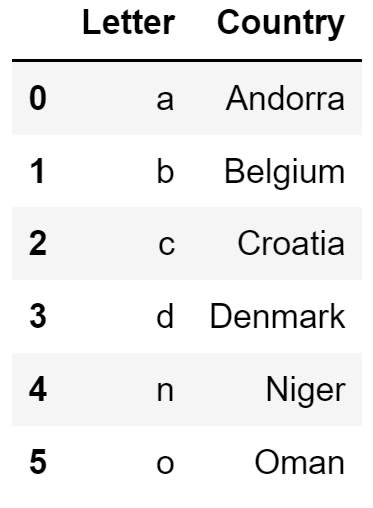Image Source: Edlitera

And this is what my second DataFrame looks like: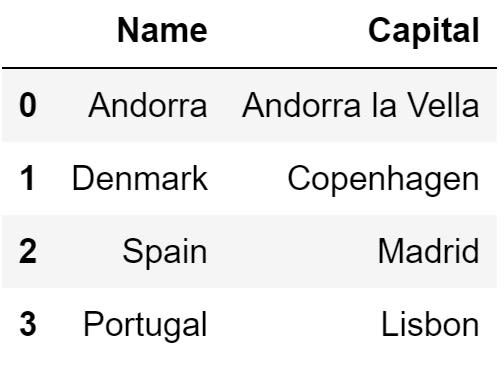Image Source: Edlitera

Article continues below

## How to Left Merge a Pandas DataFrame

The first merge I am going to take a look at is the left merge. This procedure creates a new DataFrame object by combining data from two DataFrames in such a way that all rows from the left-hand side DataFrame are included, while the rows from the right-hand-sided one are included only if they match

It functions very similarly to a left outer join for those of you familiar with SQL.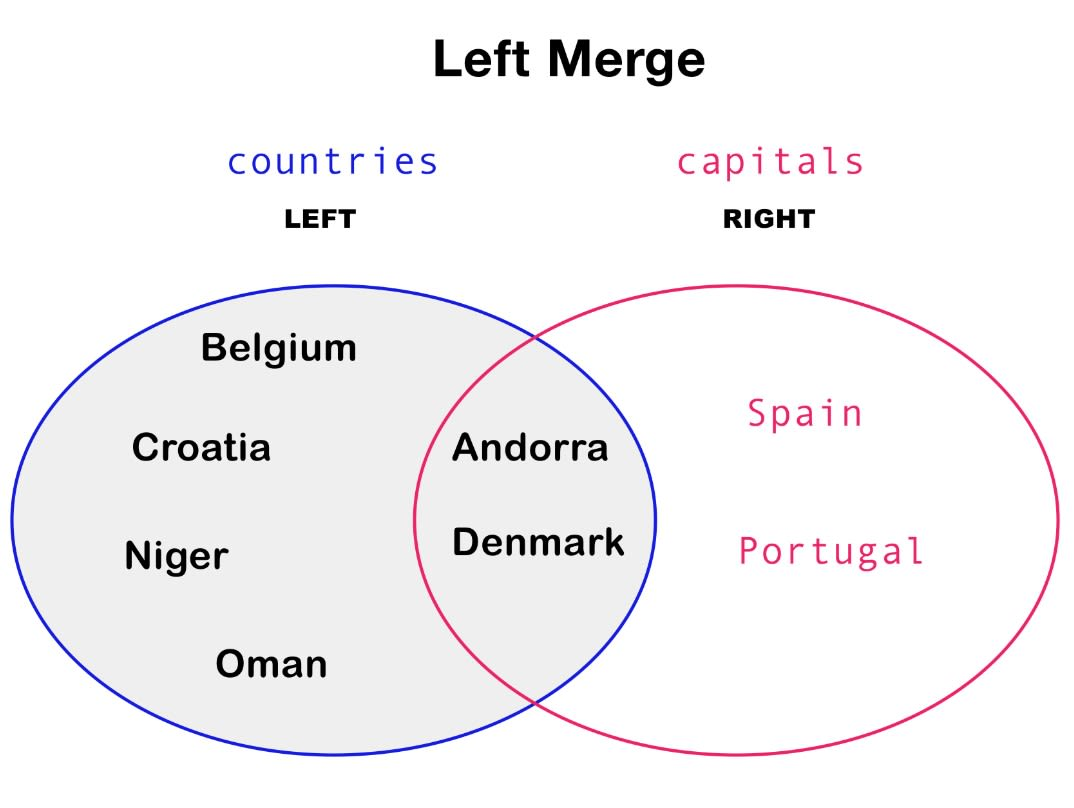Image Source: Edlitera

To perform a left merge, you use the merge() method from Pandas. You use this method to perform not only the left merge, but any other type of merge procedure.

The merge() method requires you to input values that define:

• The first DataFrame
• The second DataFrame
• Which one of them is the left-hand side DataFrame
• Which one of them is the right-hand side DataFrame
• The type of merge we want to perform

So to perform a left merge with your two DataFrames, you are going to use the following code:

``````# Perform a left merge
pd.merge(
countries, capitals,
left_on='Country', right_on='Name',
how='left'
)``````

The DataFrame that you get as the result of this process will look like this: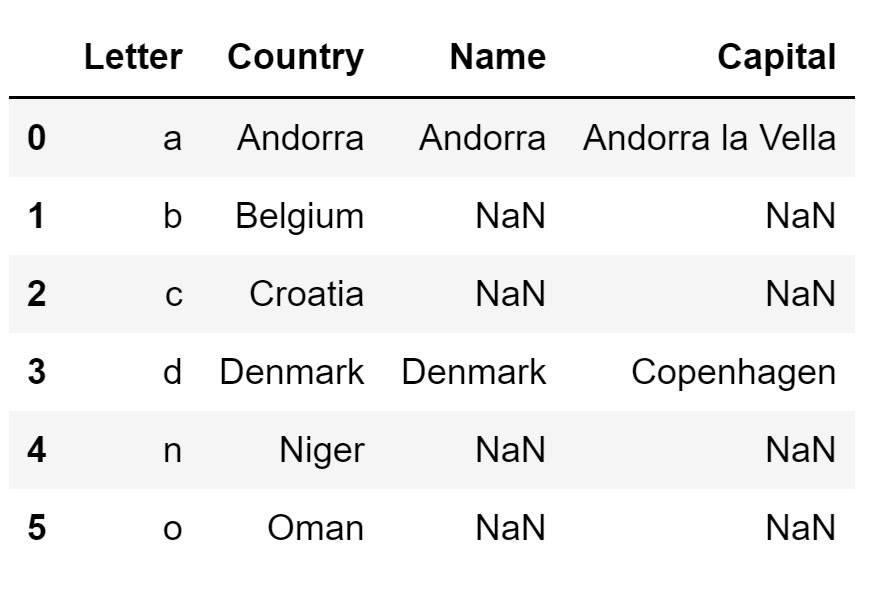Image Source: Edlitera

As you can see, there are some NaN values present in the DataFrame. The reason for that is very simple. You have appropriate values from the right-hand side DataFrame only for Denmark and Andorra. The other two countries in the right-hand side DataFrame are Spain and Portugal - because the right-hand side DataFrame doesn't contain values for Niger, Oman, Belgium, and Croatia, you are going to end up with missing values in your final DataFrame.

## How to Right Merge a Pandas DataFrame

The right merge procedure is essentially a mirrored version of the left merge procedure.  It will create a new DataFrame that includes all rows from the right-hand side DataFrame, and only those rows from the left-hand side DataFrame that match

It functions very similarly to a right outer join for those of you familiar with SQL.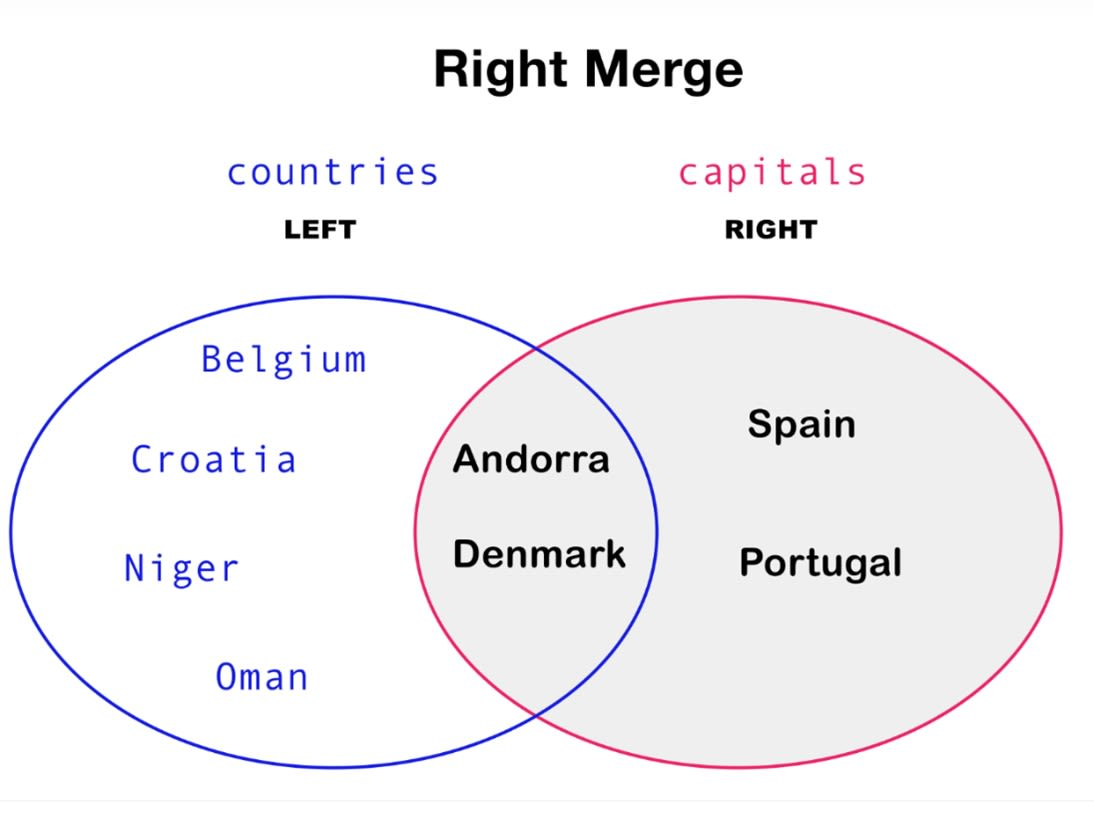Image Source: Edlitera

To perform the right merge I'm going to use the following code:

``````# Perform a right merge
pd.merge(
countries, capitals,
left_on='Country', right_on='Name',
how='right'
)``````

If you perform the right merge, you are going to end up creating the following DataFrame: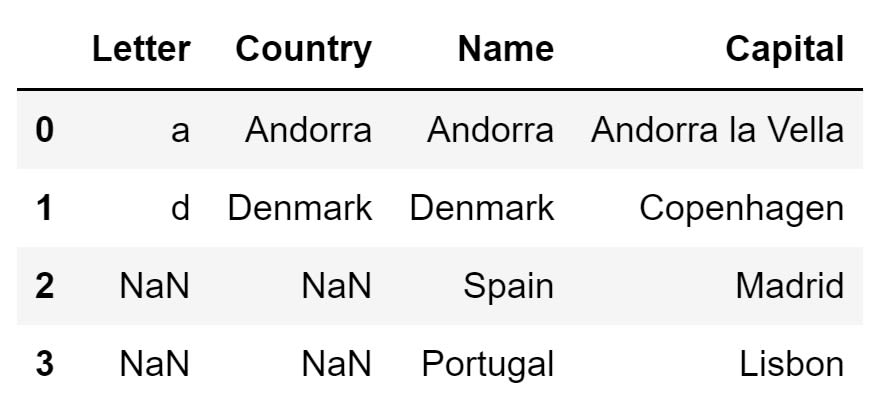Image Source: Edlitera

## How to Inner Merge a Pandas DataFrame

The inner merge procedure will create a new DataFrame that includes only those rows that have key values present in both DataFrames. That means that you can't end up with missing values.

It functions very similarly to an inner join for those of you familiar with SQL.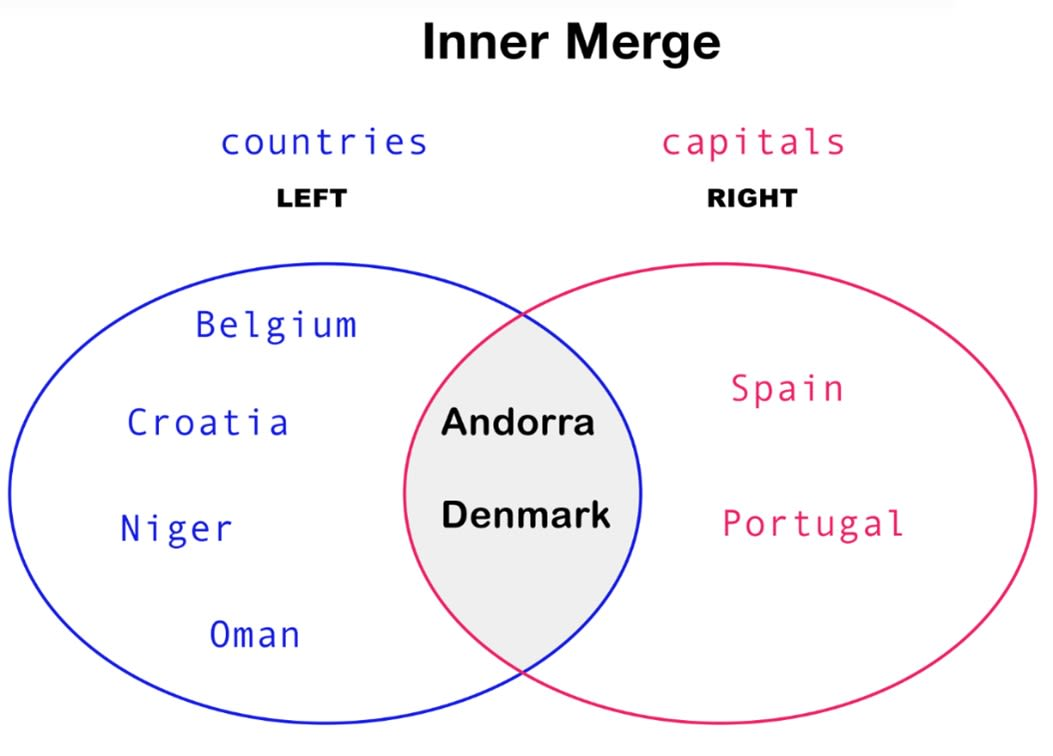Image Source: Edlitera

To perform the inner merge, you are going to use the following code:

``````# Perform an inner merge
pd.merge(
countries, capitals,
left_on='Country', right_on='Name',
how='inner'
)``````

If you perform the inner merge, you will end up creating the following DataFrame: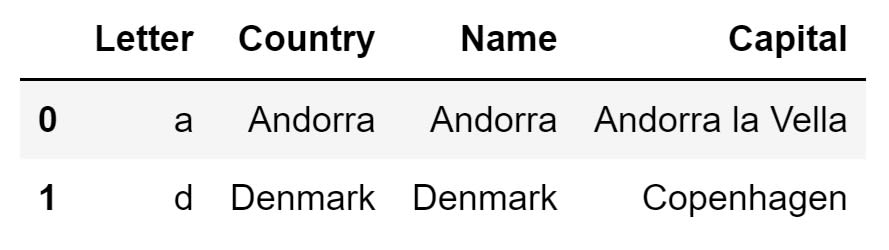Image Source: Edlitera

## How to Outer Merge a Pandas DataFrame

The outer merge procedure will create a new DataFrame that includes all rows from both DataFrames. That means that you'll usually end up with a lot of missing values

It functions very similarly to a full outer join for those of you familiar with SQL.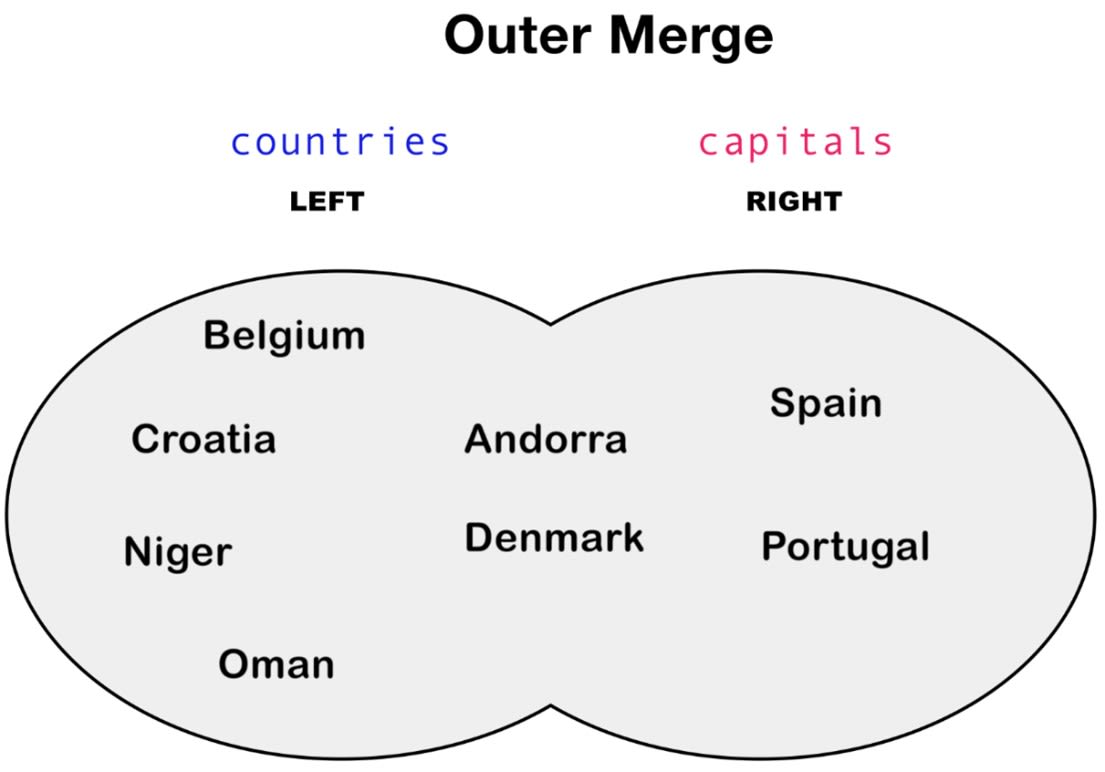Image Source: Edlitera

To perform the outer merge, you are going to use the following code:

``````# Perform an outer merge
pd.merge(
countries, capitals,
left_on='Country', right_on='Name',
how='outer'
)``````

If you perform the outer merge, you are going to end up creating the following DataFrame: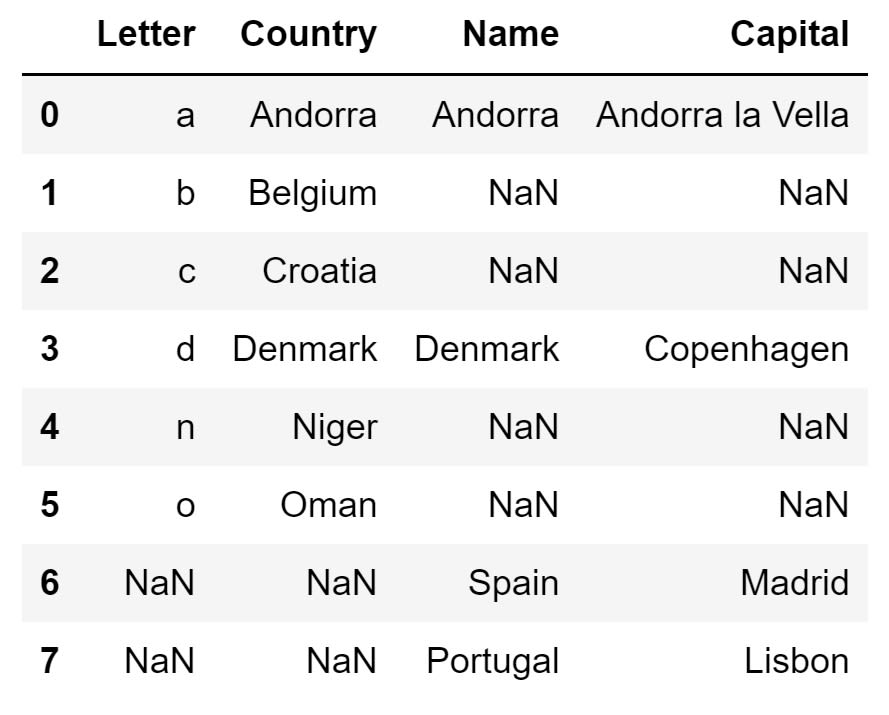Image Source: Edlitera

In this article, I covered how to perform different types of merges in Pandas. Using the merge() method, I demonstrated how to combine two DataFrames into one. Depending on what you exactly want, I demonstrated how to combine DataFrames in different ways. By mastering the different types of merges that can be performed using the merge() method, you will be ready to use data from multiple sources in your workflow.# Boris Delovski

Boris is a data science trainer and consultant who is passionate about sharing his knowledge with others.

Before Edlitera, Boris applied his skills in several industries, including neuroimaging and metallurgy, using data science and deep learning to analyze images.